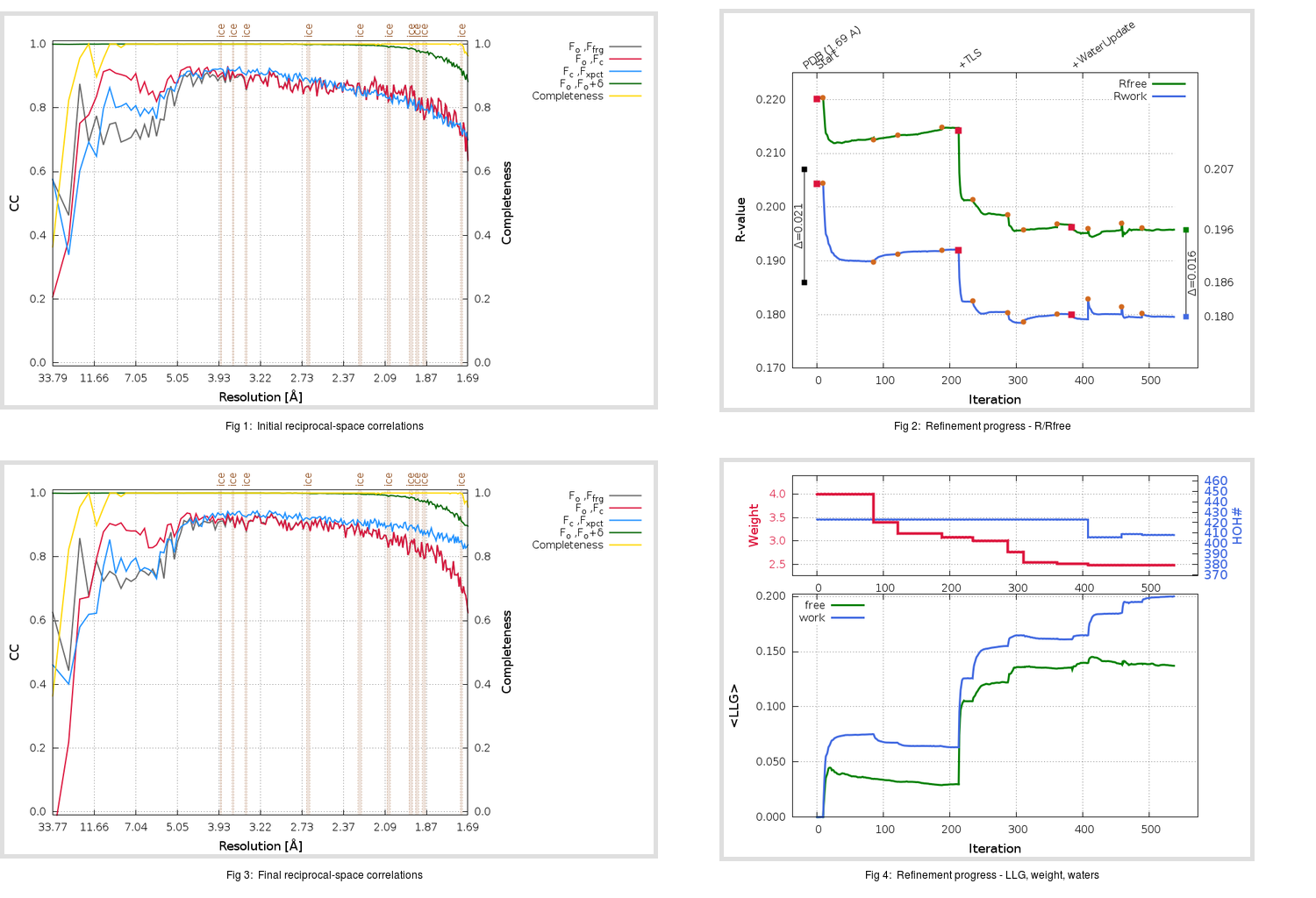Content:

```    Diffraction limits & principal axes of ellipsoid fitted to diffraction cut-off surface:
1.666         0.9497   0.0000   0.3132       0.999 a* + 0.035 c*
1.655         0.0000   1.0000   0.0000       b*
1.628        -0.3132   0.0000   0.9497      -0.537 a* + 0.844 c*
```

## Deposited

` `
 Date deposited Date data collection Resolution R, Rfree 20110719 20100806 1.69 0.1850 0.2070

Molprobity (CCP4 7.0 version) summary:

```Ramachandran outliers =   0.00 %
favored =  98.36 %
Rotamer outliers      =   0.00 %
C-beta deviations     =     1
Clashscore            =   4.36
RMS(bonds)            =   0.0099
RMS(angles)           =   1.23
MolProbity score      =   1.22
Resolution            =   1.69
R-work                =   0.1850
R-free                =   0.2070
```

```Number of waters      =   423

<B> (all atoms) =   25.60 ( sd =    8.10 ) for       2854 non-hydrogen atoms
<B>   (protein) =   24.56 ( sd =    7.22 ) for       2389 non-hydrogen atoms
<B>     (water) =   31.72 ( sd =    9.70 ) for        423 non-hydrogen atoms
<B>    (others) =   22.40 ( sd =    9.20 ) for         42 non-hydrogen atoms

B min/max       (all non-hydrogen atoms) =   12.58 /   58.08
B min/max   (protein non-hydrogen atoms) =   13.62 /   56.48
B min/max     (water non-hydrogen atoms) =   13.04 /   58.08
B min/max     (other non-hydrogen atoms) =   12.58 /   34.99
```

## BUSTER (re-)refinement

` `

Molprobity (CCP4 7.0 version) summary:

```Ramachandran outliers =   0.00 %
favored =  97.37 %
Rotamer outliers      =   0.37 %
C-beta deviations     =     0
Clashscore            =   3.94
RMS(bonds)            =   0.0126
RMS(angles)           =   1.55
MolProbity score      =   1.30
Resolution            =   1.69
R-work                =   0.1796
R-free                =   0.1958
```

```Number of waters      =   408

<B> (all atoms) =   28.13 ( sd =   10.61 ) for       2839 non-hydrogen atoms
<B>   (protein) =   25.95 ( sd =    8.74 ) for       2389 non-hydrogen atoms
<B>     (water) =   40.93 ( sd =   11.49 ) for        408 non-hydrogen atoms
<B>    (others) =   27.34 ( sd =    9.14 ) for         42 non-hydrogen atoms

B min/max       (all non-hydrogen atoms) =   13.95 /   74.29
B min/max   (protein non-hydrogen atoms) =   13.95 /   74.29
B min/max     (water non-hydrogen atoms) =   16.90 /   73.17
B min/max     (other non-hydrogen atoms) =   18.90 /   37.89
```

Refinement progression:Results:

` `
 File Remark 3SZN_aB_refine.01_03_refine.pdb.gz exact refinement commands are in header 3SZN_aB_refine.01_03_refine.mtz.gz including original deposited data and several re-refinement map coefficients 3SZN_aB_refine.01_03_BUSTER_model.cif.gz including any non-standard compound restraints 3SZN_aB_refine.01_03_BUSTER_refln.cif.gz